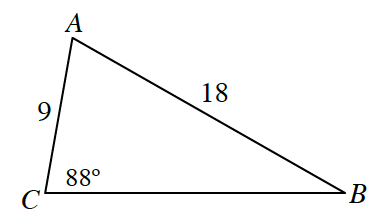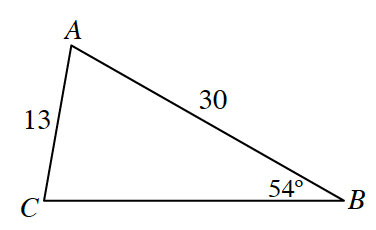### Home > PC3 > Chapter 7 > Lesson 7.2.4 > Problem7-119

7-119.

Solve each triangle below. Homework Help ✎

The given information in both of these triangles is $\text{SSA}$, so use the Law of Cosines to determine if there are $0$, $1$, or $2$ possible triangles.

1.$18^2=9^2+a^2-2(9)(a)\cos(88°)$

1.$13^2=30^2+a^2-2(30)(a)\cos(542°)$